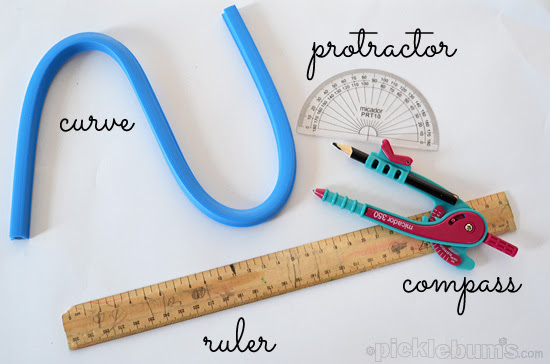## 22 August 2014

### Basic Constructions

The tools in our geometry box are:• Ruler
• Compass
• Divider
• Set squares
• Protractor
The description of each tool and its uses are given below:

Ruler:

A ruler is a flat and straight-edged strip, whose one side is graduated into centimetres and the other into inches. A ruler is commonly called a scale. It is the most essential tool in geometry. It is used in all constructions.
The basic uses of a ruler are:

Measuring lengths of line segments

Drawing line segments

Compass:

A compass has two ends. One end holds a pointer, while the other end holds a pencil. It is also called a pair of compasses.

The basic uses of a compass are:
• Marking off equal lengths
• Drawing arcs
• Drawing circle
Divider:

A divider is a tool similar in shape to a compass. It has a pair of pointer ends.

The basic uses of a divider are:
• Comparing lengths of line segments
• Helping avoid positioning errors
• Taking accurate measurements
Set squares:

The two triangular tools in the geometry box are called set squares. One of the set square is an isosceles triangle with two angles measuring 45° each. The other set square is a scalene triangle with two angles measuring 30° and 60° each. The two perpendicular sides of either set square are graduated into centimetres.

The basic uses of set squares are:
• Drawing perpendicular lines
• Drawing parallel lines
Protractor:

A semi-circular tool with degrees marked is called a protractor. The centre of the semicircle is called the midpoint of the protractor. This point helps as a reference point for the protractor. The horizontal line is called the base line or the straight edge of the protractor.

The basic uses of a protractor are:
• Measuring angles
• Drawing angles
The important points to be remembered while using the tools for construction are:
• Draw smooth and thin lines
• Mark points lightly
• Maintain tools or instruments with sharp pointers and fine edges
• Keep two pencils in the box. One is for drawing lines and marking points. The other is for using in the compass

### Construction of  Angels

 With our free PDF notes you can get success in any competitive or entrance exams like CTET,  KVS, NET, CAT, MAT, CMAT, SSC, B.ED, IBPS Recruitment, IAS, CSAT, State Civil Services Exams, UPTET, PSTET, HTET & many more. It also provides NCERT solutions, CBSE, NTSE, Olympiad study material, Indian General Knowledge, English Grammar, Hindi,  Mathematics Tricks, Current affairs, Science, S.ST, model test papers, important Question.